# python tutorial - Python interview questions pdf - learn python - python programming

## Dictionary of types?

• Since type names don't have to be unique across modules, you must use the type (classObject) itself.
• Luckily, types will automatically have a non-trivial hash value: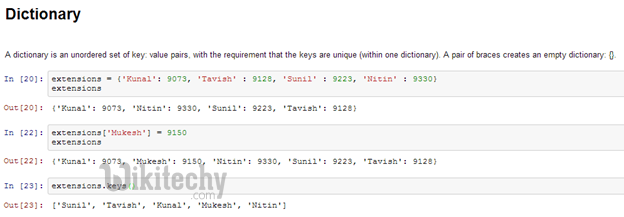Learn python - python tutorial - python-dictionary - python examples - python programs

## What is the purpose of the `//` operator in python?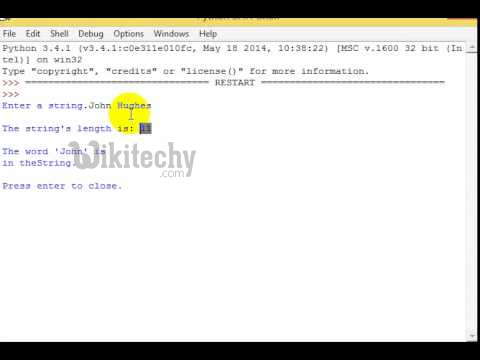Learn python - python tutorial - operator-in-python - python examples - python programs

Integer division vs. float division:

## What Is The Command To Debug A Python Program?

• The following command helps run a Python program in debug mode.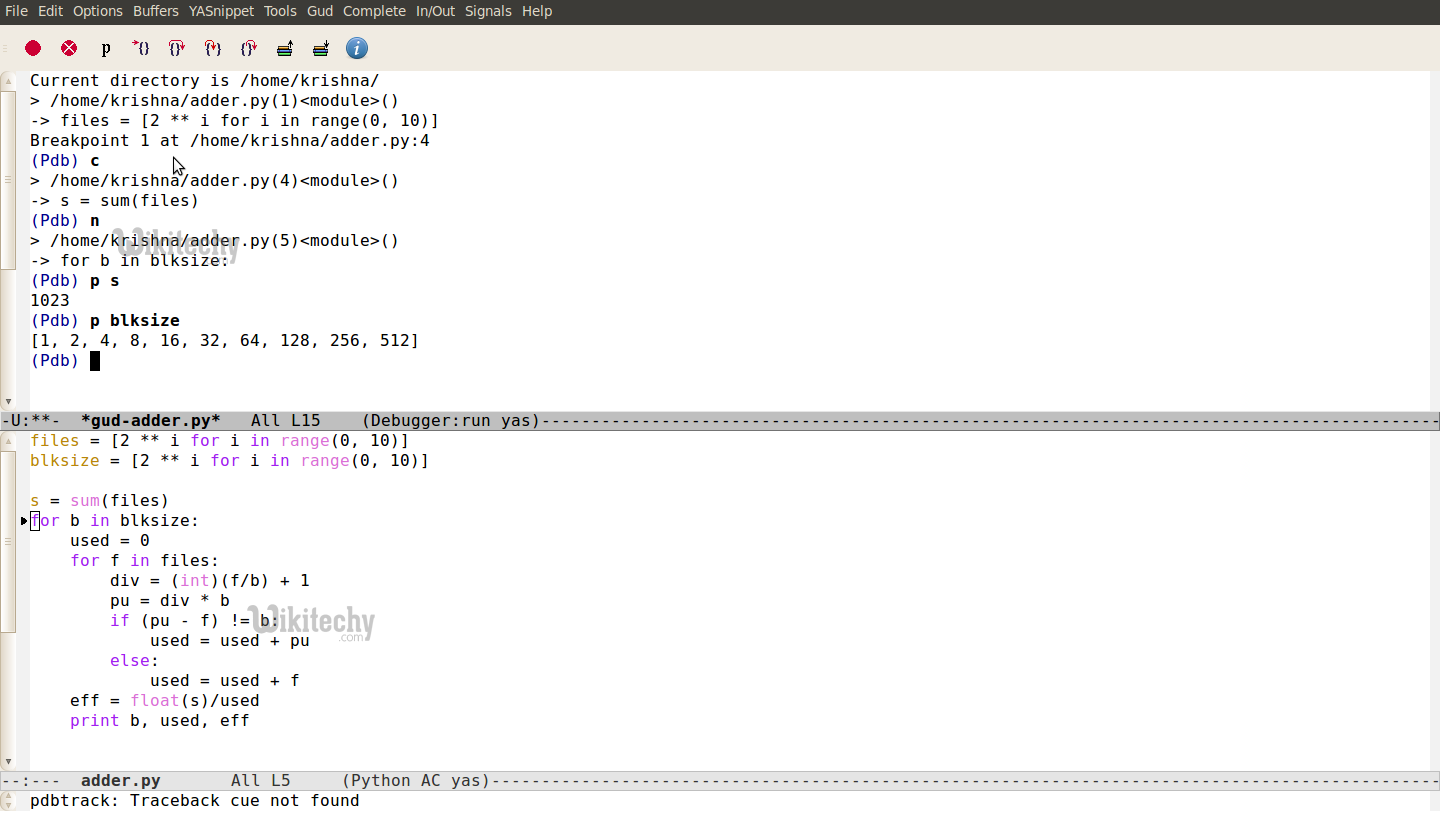Learn python - python tutorial - command-to-debug-python-program - python examples - python programs

## How Do You Monitor The Code Flow Of A Program In Python?

• In Python, we can use <sys> module’s <settrace()> method to setup trace hooks and monitor the functions inside a program.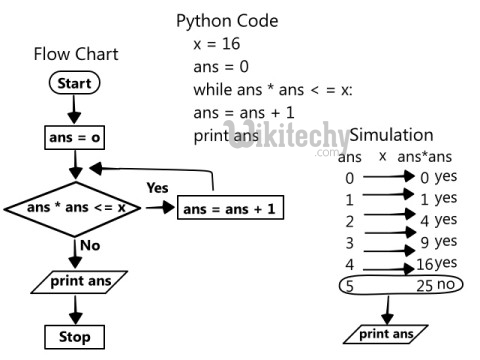Learn python - python tutorial - python-flow-chart - python examples - python programs

• You need to define a trace callback method and pass it to the <settrace()> method. The callback should specify three arguments as shown below.

## Why And When Do You Use Generators In Python?

• A generator in Python is a function which returns an iterable object. We can iterate on the generator object using the <yield> keyword. But we can only do that once because their values don’t persist in memory, they get the values on the fly.
• Generators give us the ability to hold the execution of a function or a step as long as we want to keep it. However, here are a few examples where it is beneficial to use generators.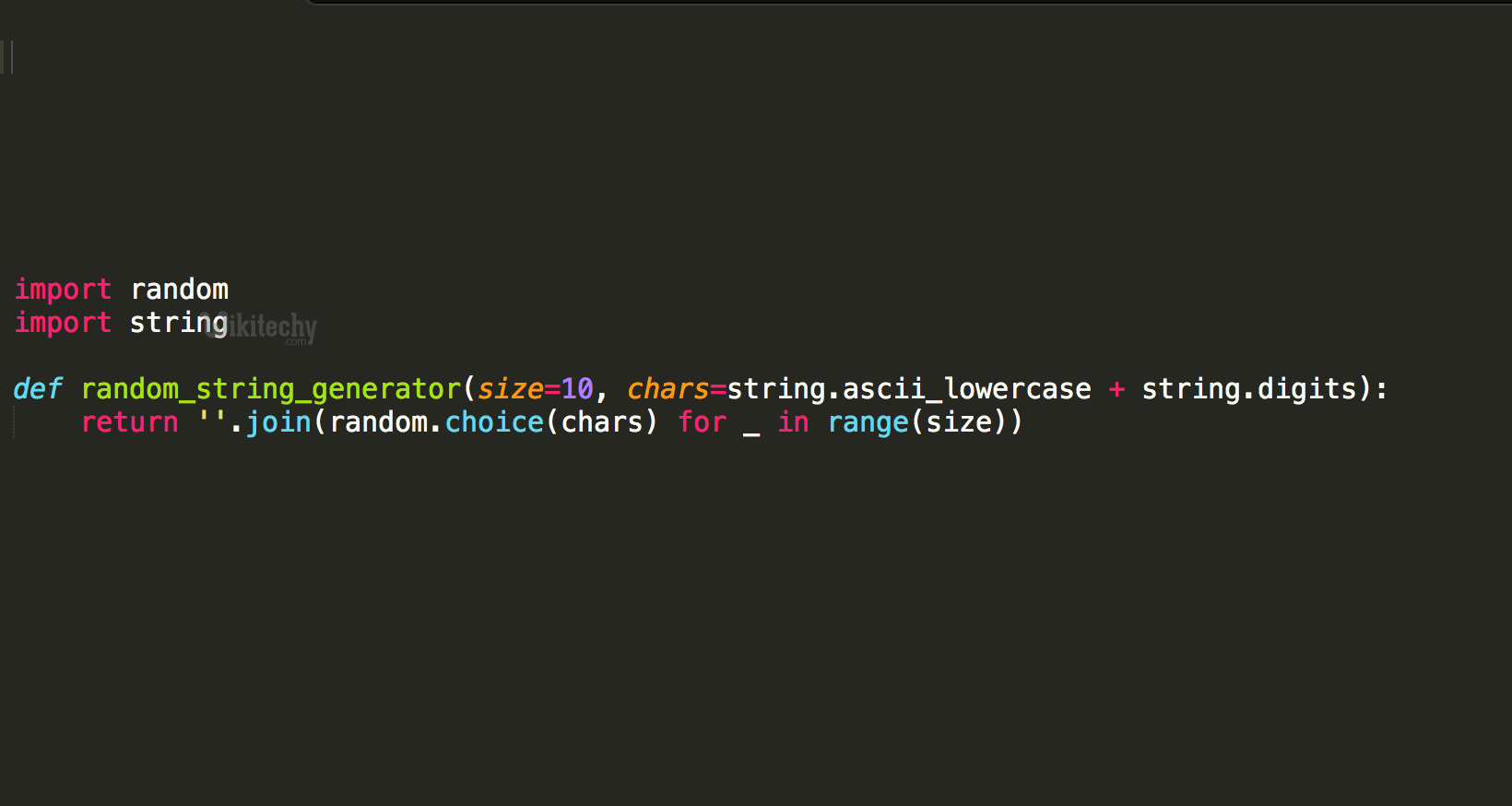Learn python - python tutorial - generators-in-python - python examples - python programs

• We can replace loops with generators for efficiently calculating results involving large data sets.
• Generators are useful when we don’t want all the results and wish to hold back for some time.
• Instead of using a callback function, we can replace it with a generator. We can write a loop inside the function doing the same thing as the callback and turns it into a generator.

## What Does The <Yield> Keyword Do In Python?

• The <yield> keyword can turn any function into a generator. It works like a standard return keyword. But it’ll always return a generator object. Also, a function can have multiple calls to the <yield> keyword.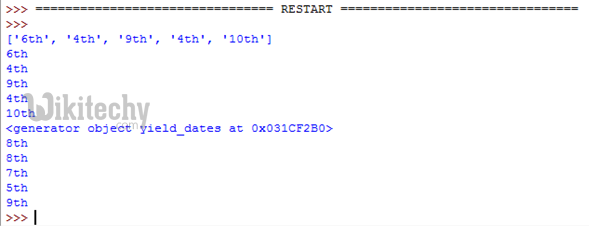Learn python - python tutorial - yield-keyword-in-python - python examples - python programs

## How To Convert A List Into Other Data Types?

• Sometimes, we don’t use lists as is. Instead, we have to convert them to other types.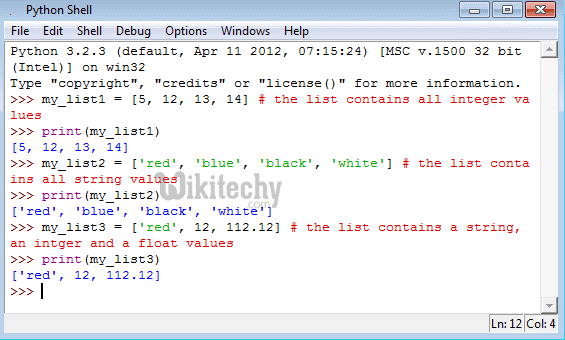Learn python - python tutorial - python-shell - python examples - python programs

## Turn A List Into A String.

• We can use the <”.join()> method which combines all elements into one and returns as a string.

## Turn A List Into A Tuple.

• Call Python’s <tuple()> function for converting a list into a tuple. This function takes the list as its argument. But remember, we can’t change the list after turning it into a tuple because it becomes immutable.

## Turn A List Into A Set.

• Converting a list to a set poses two side-effects.
• Set doesn’t allow duplicate entries, so the conversion will remove any such item if found.
• A set is an ordered collection, so the order of list items would also change.
• However, we can use the <set()> function to convert a list to a set.

## Turn A List Into A Dictionary.

• In a dictionary, each item represents a key-value pair. So converting a list isn’t as straight forward as it were for other data types.
• However, we can achieve the conversion by breaking the list into a set of pairs and then call the <zip()> function to return them as tuples.
• Passing the tuples into the <dict()> function would finally turn them into a dictionary.

## How Do You Count The Occurrences Of Each Item Present In The List Without Explicitly Mentioning Them?

• Unlike sets, lists can have items with same values. In Python, the list has a <count()> function which returns the occurrences of a particular item.Learn python - python tutorial - python-variable - python examples - python programs

• Count The Occurrences Of An Individual Item.

## output:

• Count The Occurrences Of Each Item In The List.
1. We’ll use the list comprehension along with the <count()> method. It’ll print the frequency of each of the items.

## What Is NumPy And How Is It Better Than A List In Python?

• NumPy is a Python package for scientific computing which can deal with large data sizes. It includes a powerful N-dimensional array object and a set of advanced functions.
• Also, the NumPy arrays are superior to the built-in lists. There are a no. of reasons for this.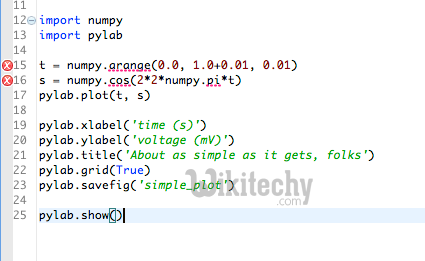Learn python - python tutorial - nympy-python - python examples - python programs

• NumPy arrays are more compact than lists.
• Reading and writing items is faster with NumPy.
• Using NumPy is more convenient than to the standard list.
• NumPy arrays are more efficient as they augment the functionality of lists in Python.

## What Are Different Ways To Create An Empty NumPy Array In Python?

• There are two methods which we can apply to create empty NumPy arrays.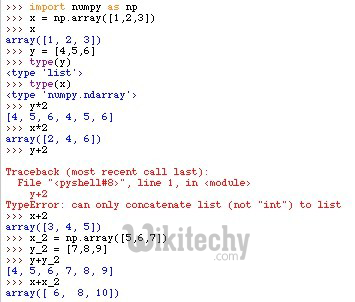Learn python - python tutorial - nympy-array-in-python - python examples - python programs

• The First Method To Create An Empty Array.
• The Second Method To Create An Empty Array.